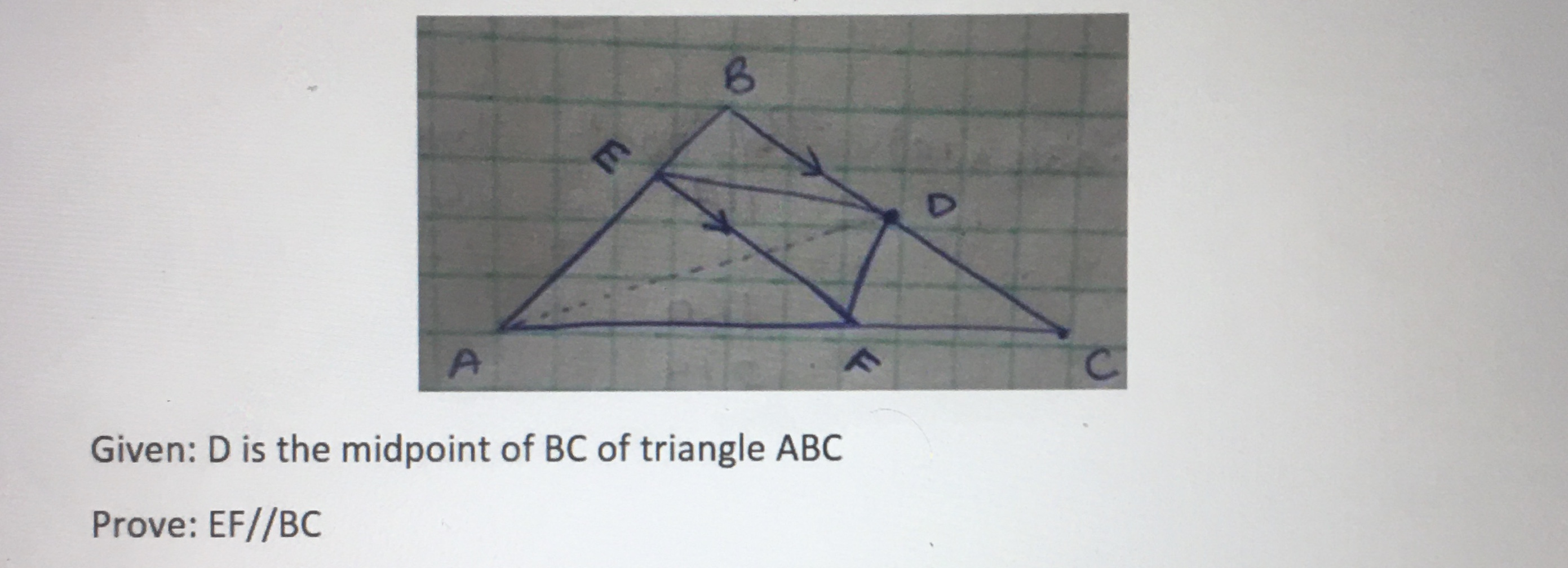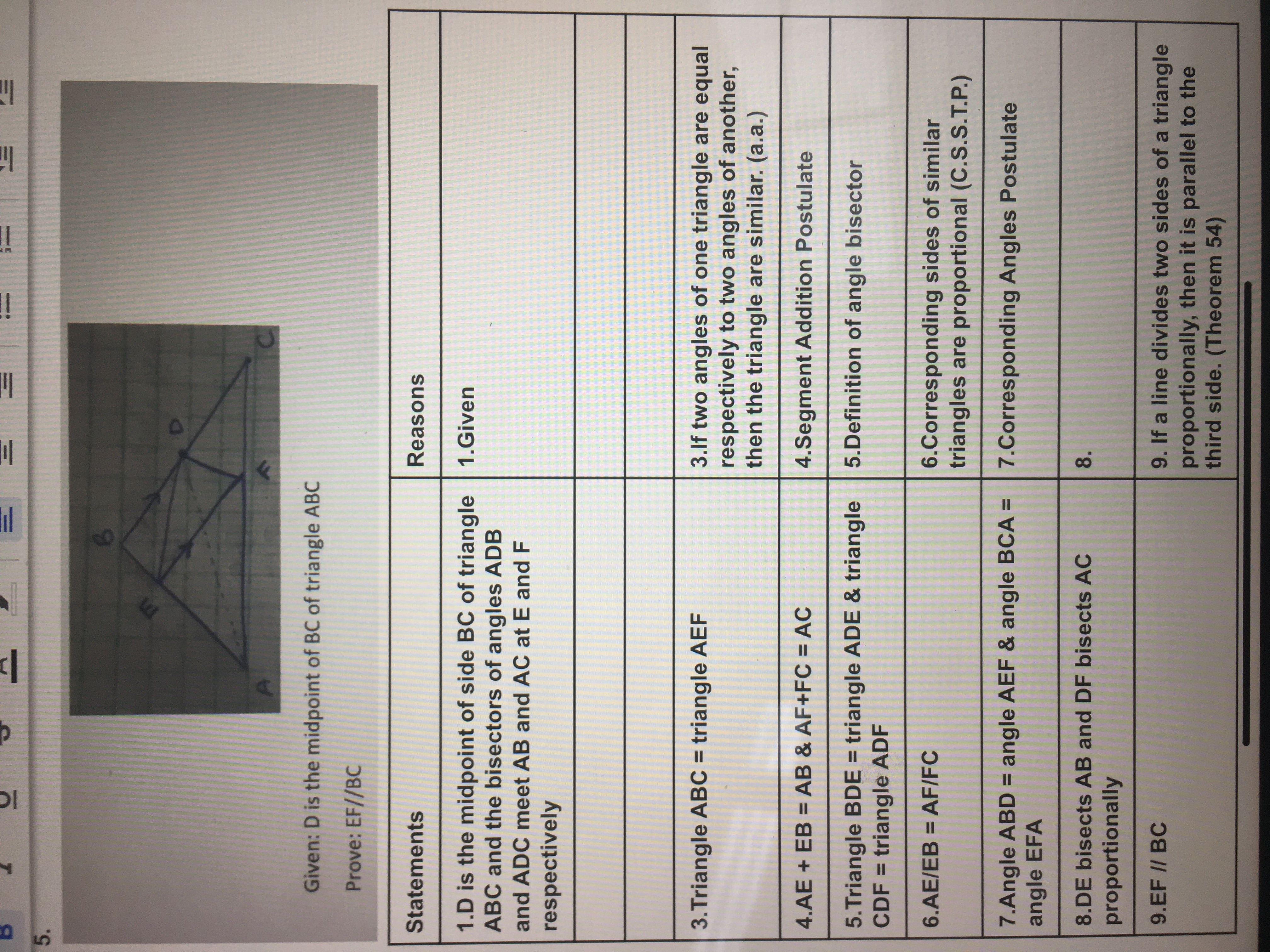# A. C. Given: D is the midpoint of BC of triangle ABC Prove: EF//BC E. 5. Given: D is the midpoint of BC of triangle ABC Prove: EF//BC Statements Reasons 1.D is the midpoint of side BC of triangle 1.Given ABC and the bisectors of angles ADB and ADC meet AB and AC at E and F respectively 3.Triangle ABC = triangle AEF 3.lf two angles of one triangle are equal respectively to two angles of another, then the triangle are similar. (a.a.) 4.AE + EB = AB & AF+FC = AC 4.Segment Addition Postulate %3D 5.Triangle BDE = triangle ADE & triangle CDF = triangle ADF 5.Definition of angle bisector %3D 6.Corresponding sides of similar triangles are proportional (C.S.S.T.P.) 6.AE/EB = AF/FC %3D 7.Angle ABD = angle AEF & angle BCA = 7.Corresponding Angles Postulate angle EFA %3D 8.DE bisects AB and DF bisects AC 8. proportionally 9. If a line divides two sides of a triangle proportionally, then it is parallel to the third side. (Theorem 54) 9.EF // BC ET !!

Questionhelp_outlineImage TranscriptioncloseA. C. Given: D is the midpoint of BC of triangle ABC Prove: EF//BC E. fullscreenhelp_outlineImage Transcriptionclose5. Given: D is the midpoint of BC of triangle ABC Prove: EF//BC Statements Reasons 1.D is the midpoint of side BC of triangle 1.Given ABC and the bisectors of angles ADB and ADC meet AB and AC at E and F respectively 3.Triangle ABC = triangle AEF 3.lf two angles of one triangle are equal respectively to two angles of another, then the triangle are similar. (a.a.) 4.AE + EB = AB & AF+FC = AC 4.Segment Addition Postulate %3D 5.Triangle BDE = triangle ADE & triangle CDF = triangle ADF 5.Definition of angle bisector %3D 6.Corresponding sides of similar triangles are proportional (C.S.S.T.P.) 6.AE/EB = AF/FC %3D 7.Angle ABD = angle AEF & angle BCA = 7.Corresponding Angles Postulate angle EFA %3D 8.DE bisects AB and DF bisects AC 8. proportionally 9. If a line divides two sides of a triangle proportionally, then it is parallel to the third side. (Theorem 54) 9.EF // BC ET !! fullscreen

### Want to see this answer and more?

Experts are waiting 24/7 to provide step-by-step solutions in as fast as 30 minutes!*

*Response times vary by subject and question complexity. Median response time is 34 minutes and may be longer for new subjects.
Tagged in
MathGeometry

### Triangles